# NCERT Exemplar Class 9 Science Solutions for Chapter 8 - Motion

## NCERT Exemplar Solutions Class 9 Science Chapter 8 – Free PDF Download

NCERT Exemplar Solution for Class 9 Science Chapter 8 Motion is prepared to help the students of Class 9 who wish to have a good foundation on the subjects, which will help them to prepare for their upcoming board and competitive exams. One such basic chapter that helps the students in their future is Motion. Here, we have provided the NCERT Exemplar for Class 9 Science Chapter 8 PDF, so that students can download the file and access the study material from anywhere in the world, at any point of time.

NCERT Exemplar Class 9 Motion offers answers to the questions provided in the NCERT Exemplar book. This Exemplar has MCQs, numerical problems, short answer questions and long answer questions. Motion is one of the most important topics in Physics. In Class 8 students will learn the basic concepts of Physics like motion in a straight line, uniform and non-uniform motion, velocity and rate of change of velocity. Enhance your learning by solving the NCERT Exemplar Science Class 9 Chapter 8 Motion. To download, visit the below link.

### Download PDF of NCERT exemplar for Class 9 Science Chapter 8 – Motion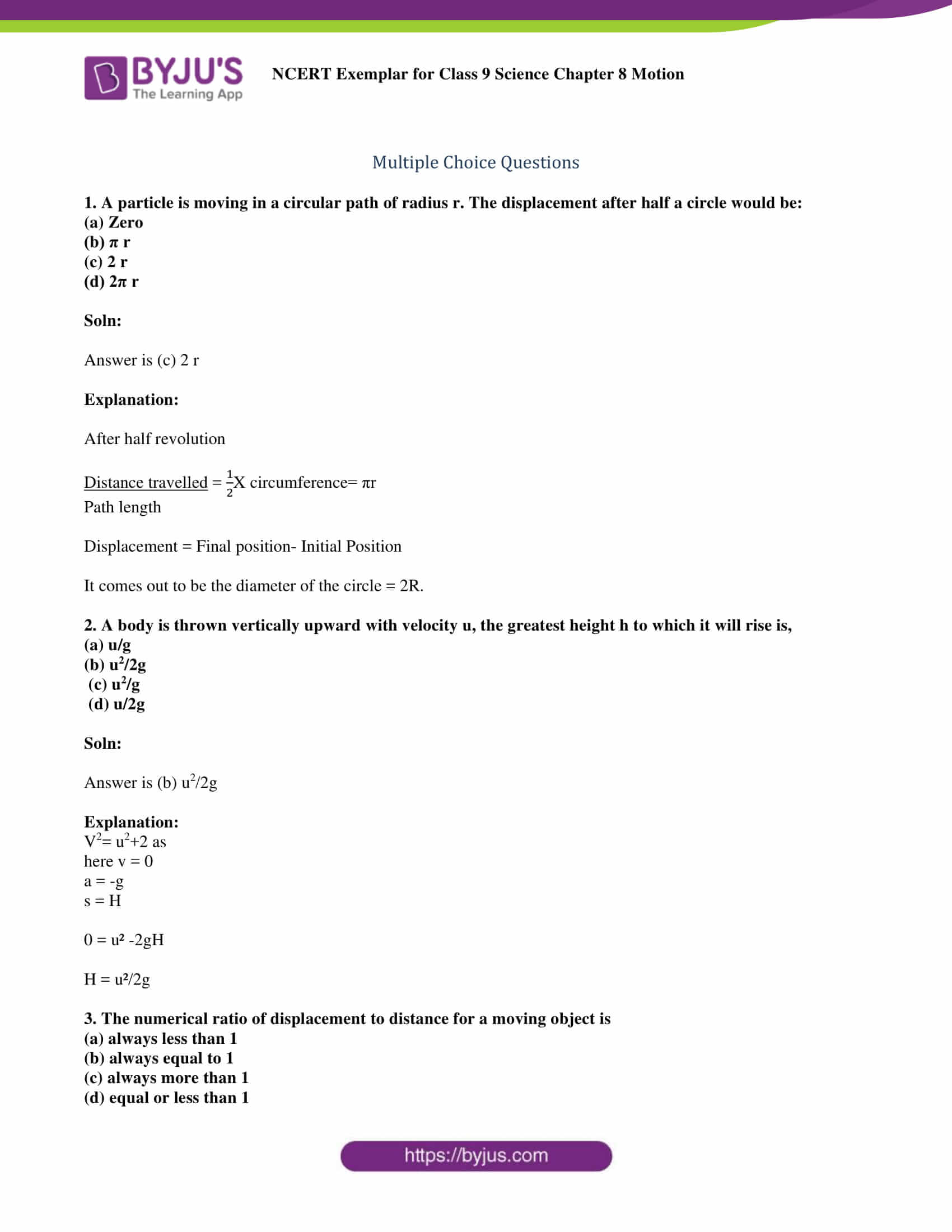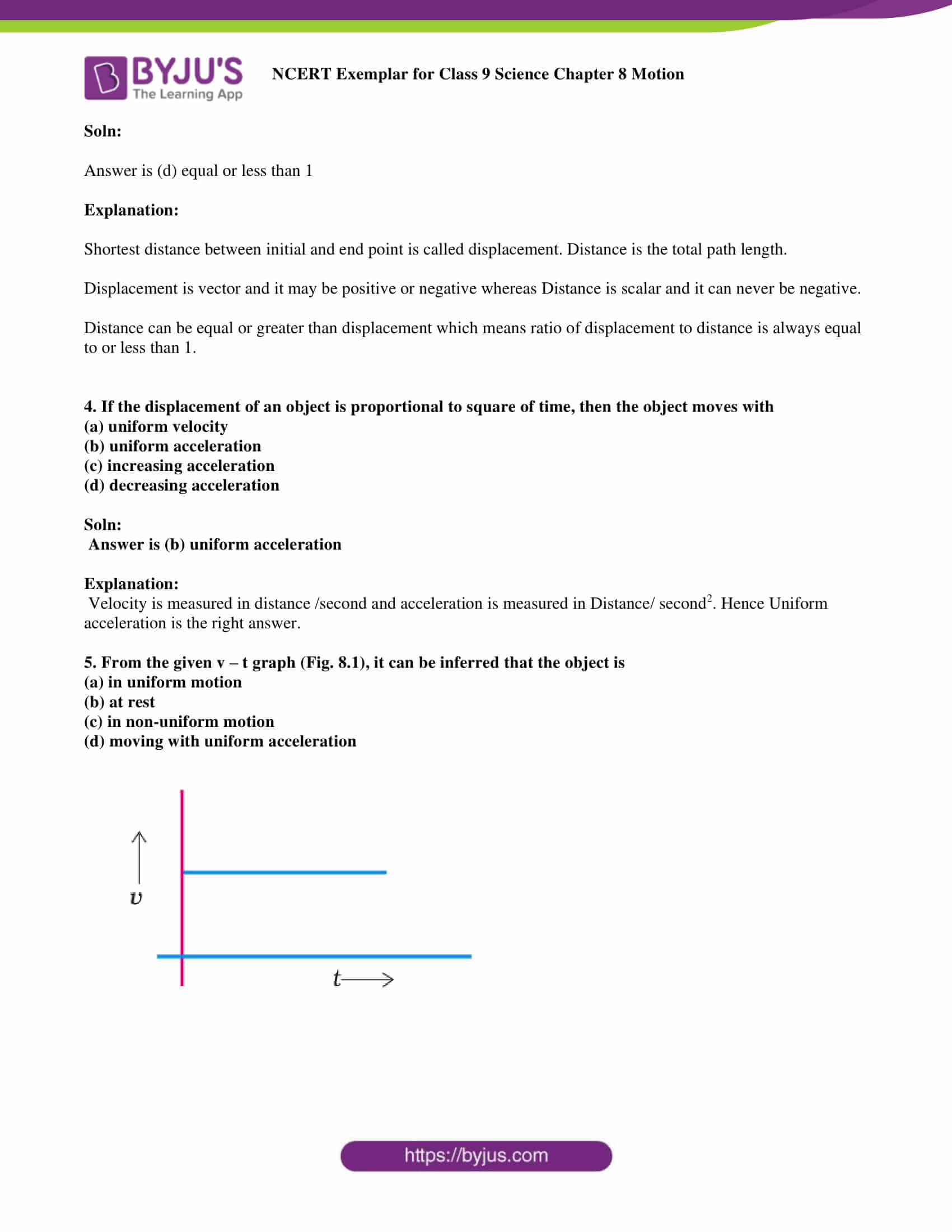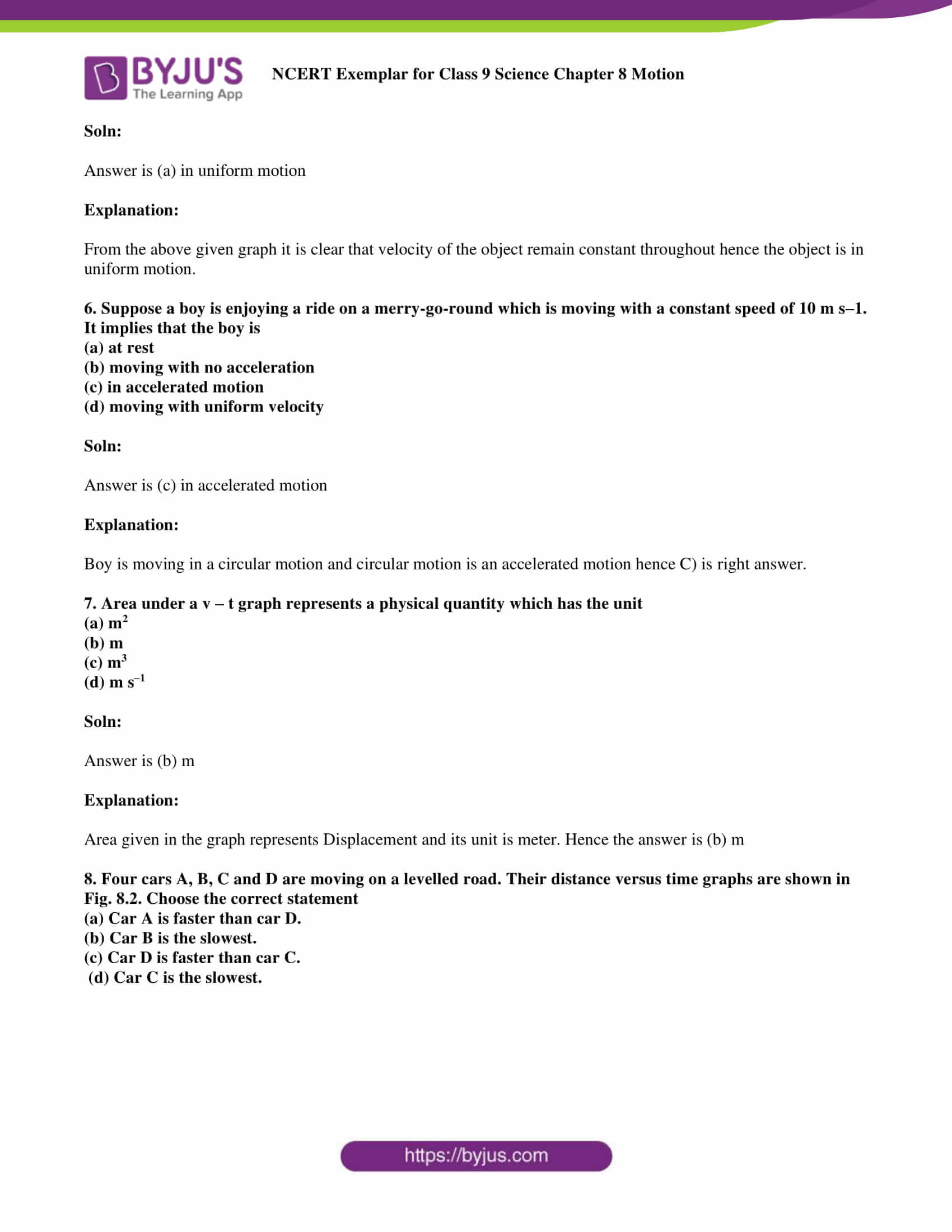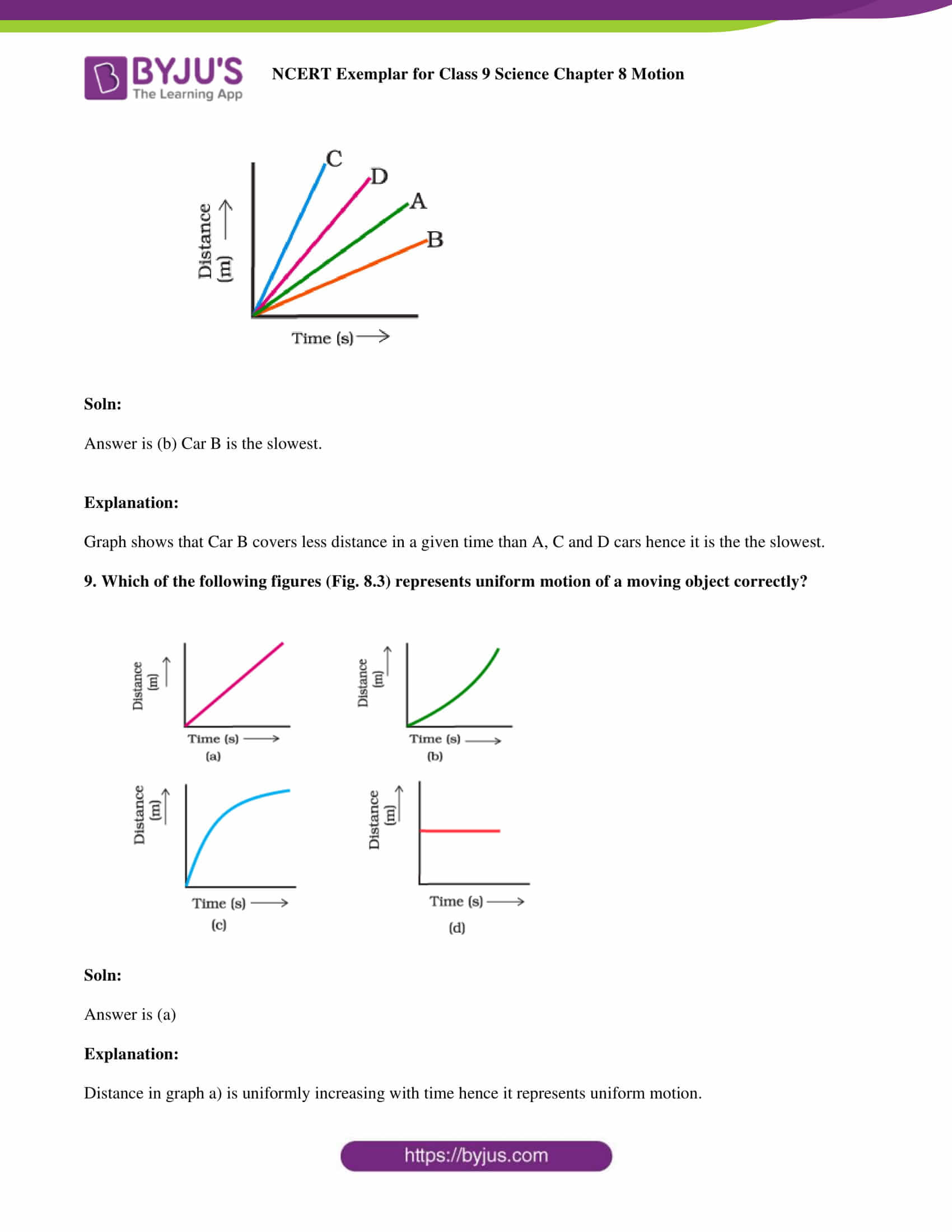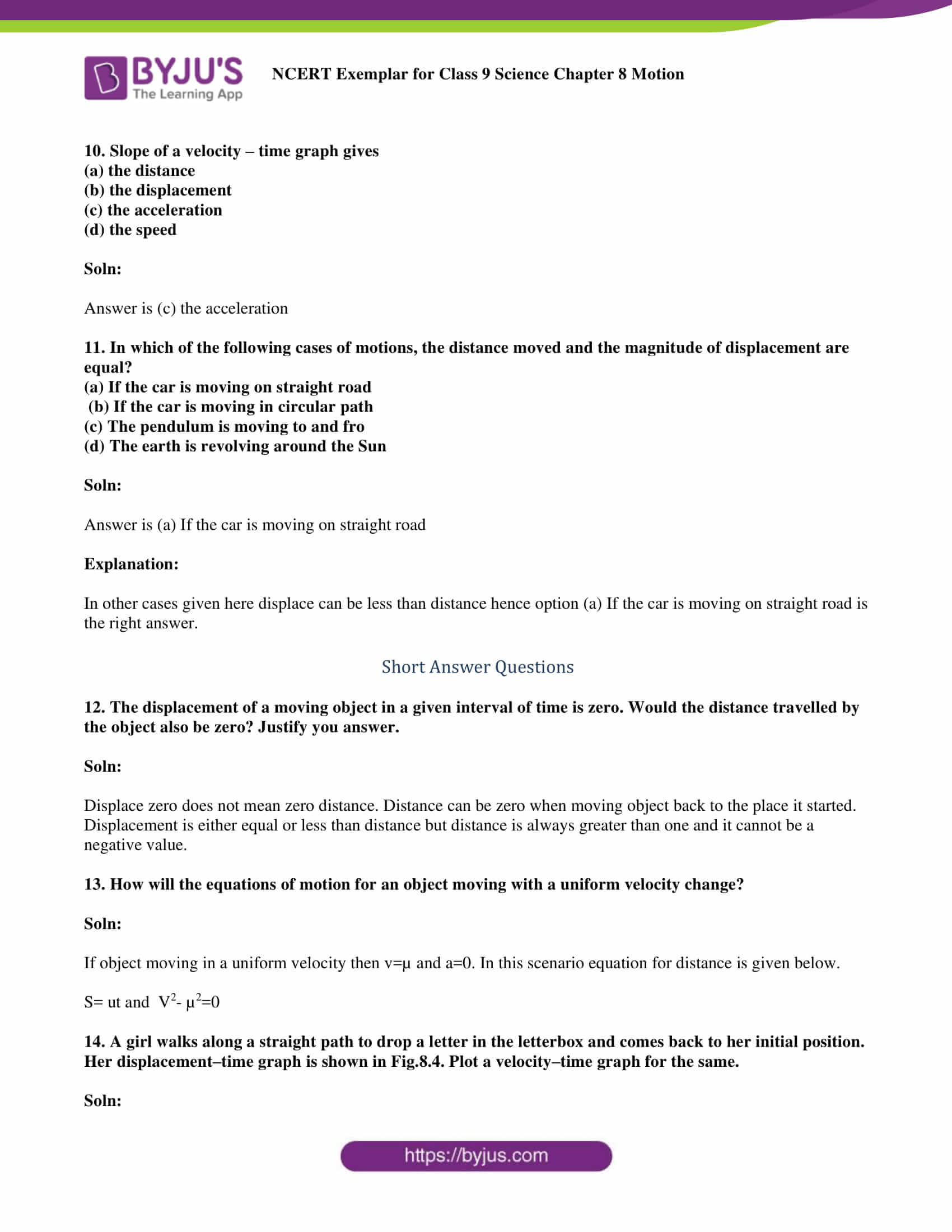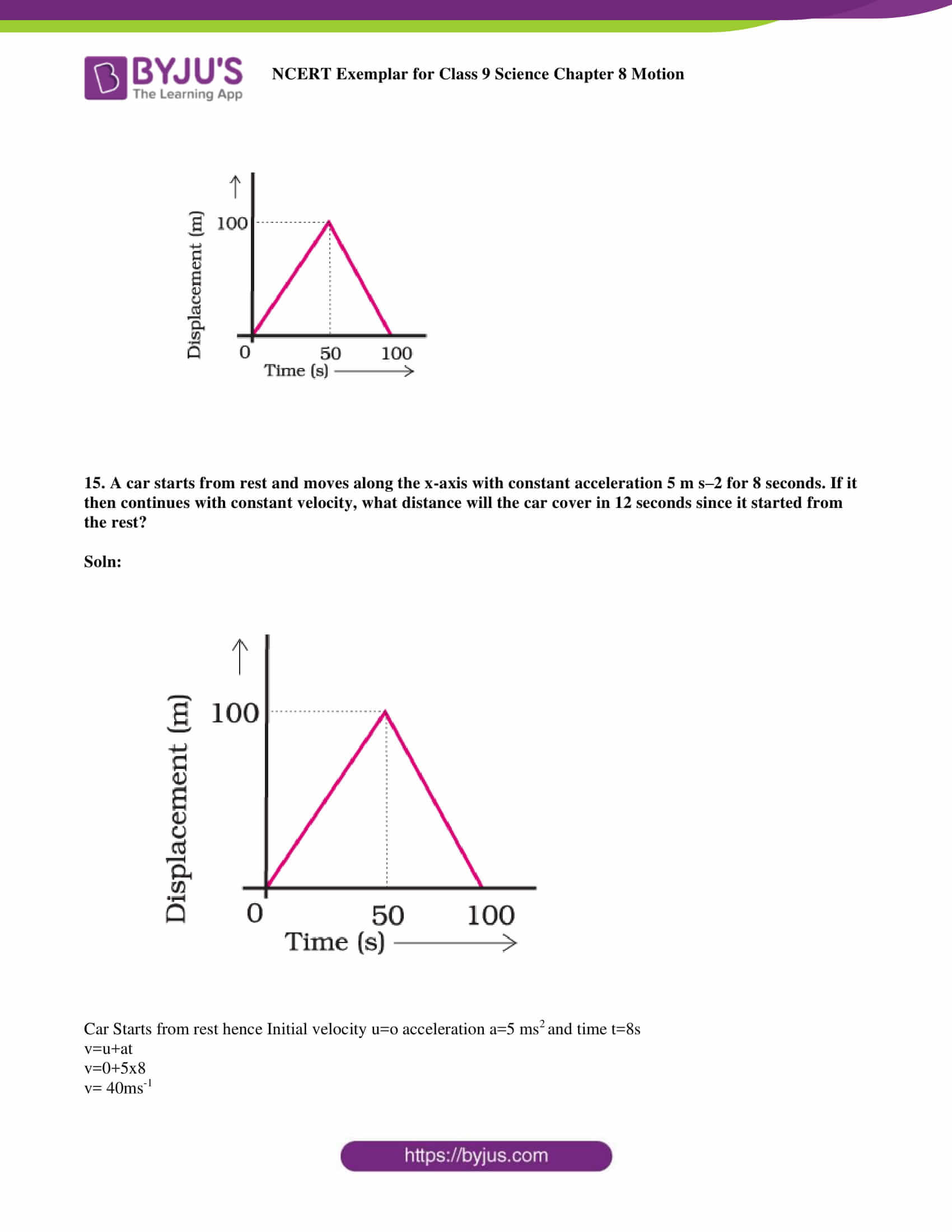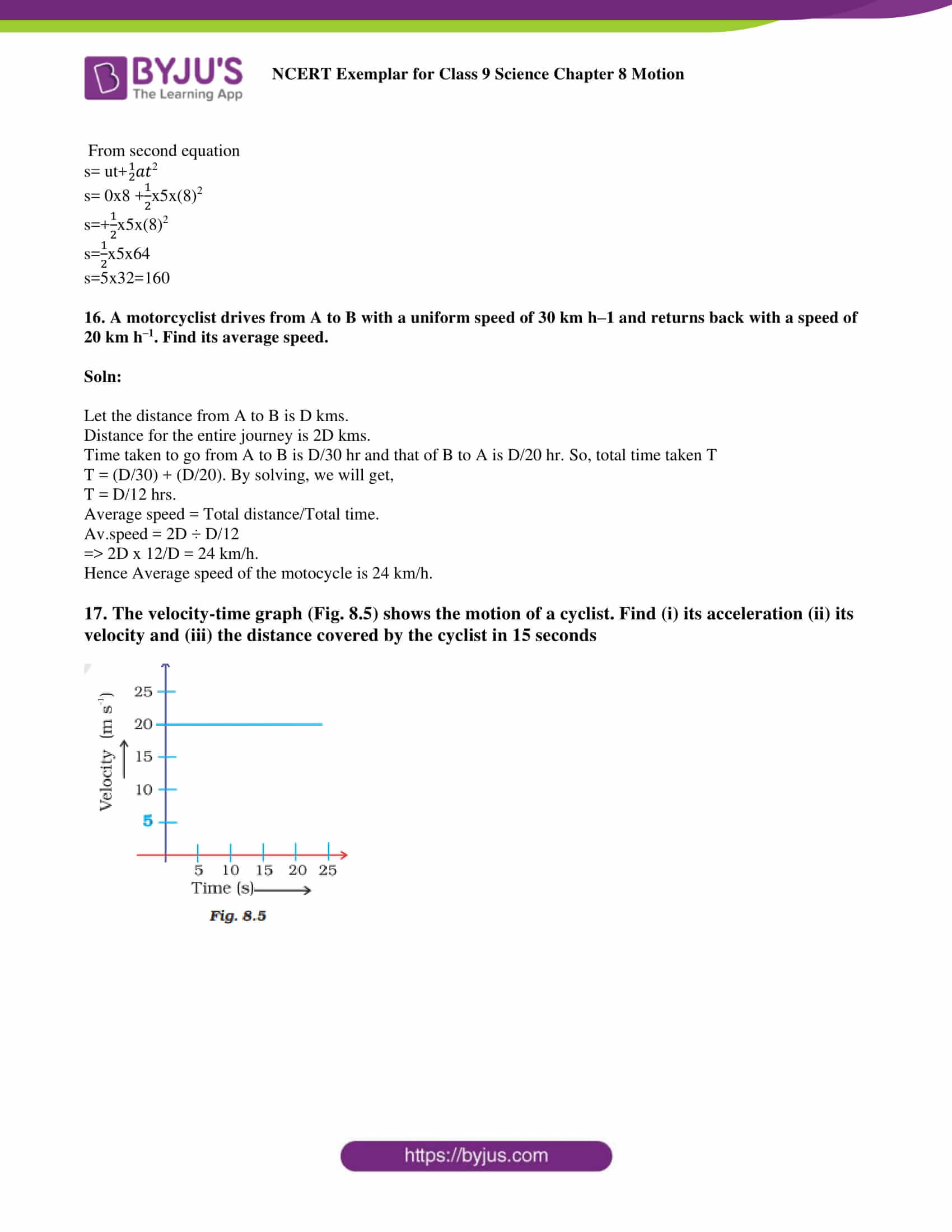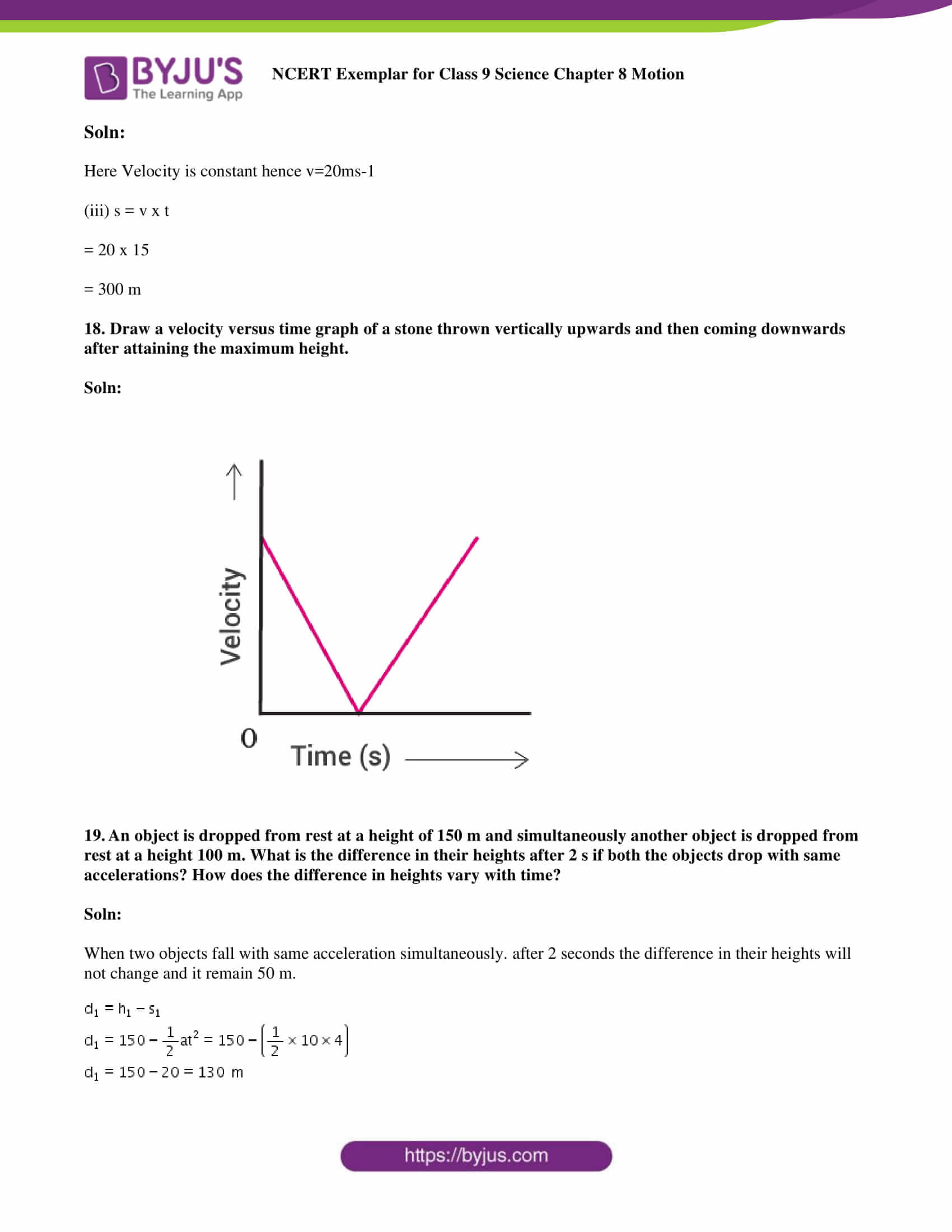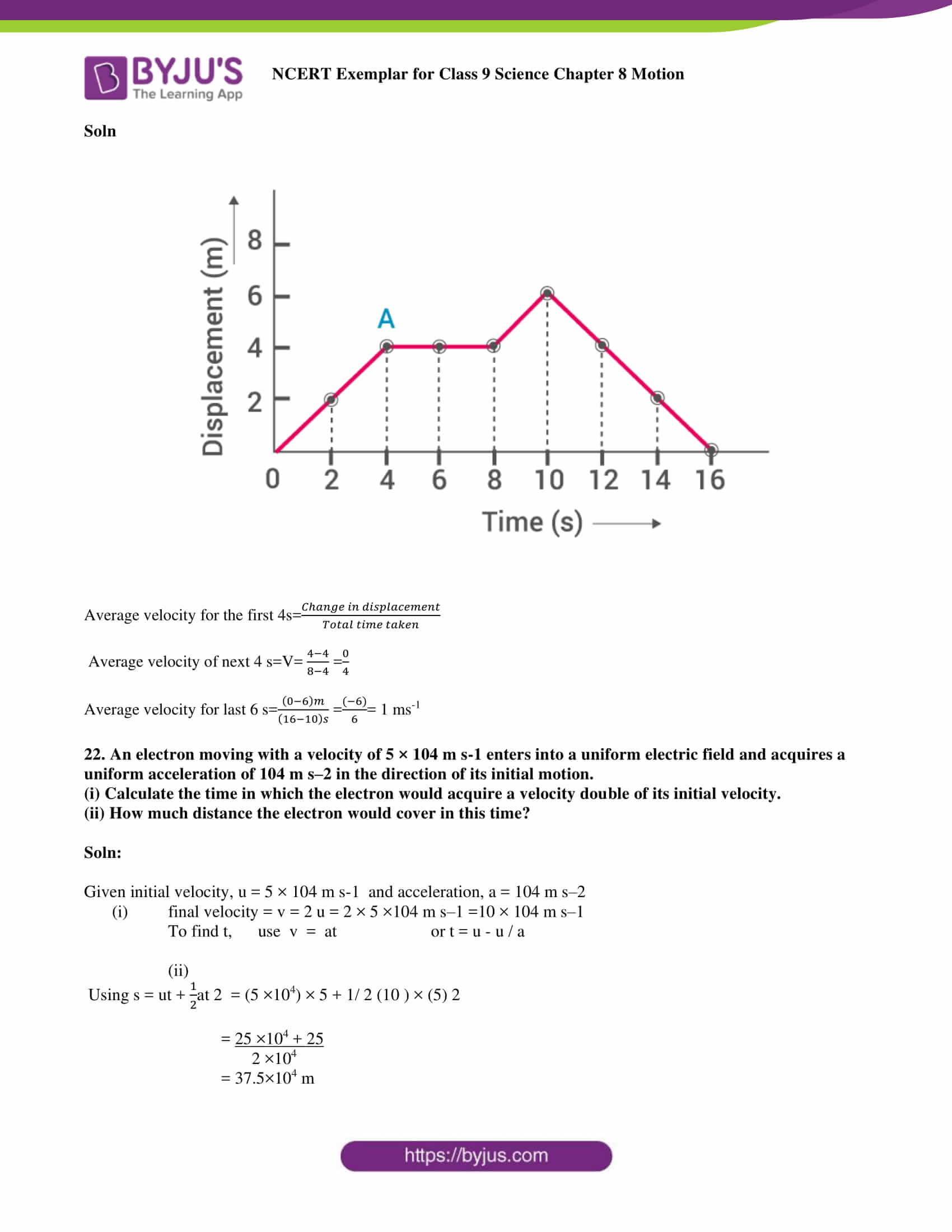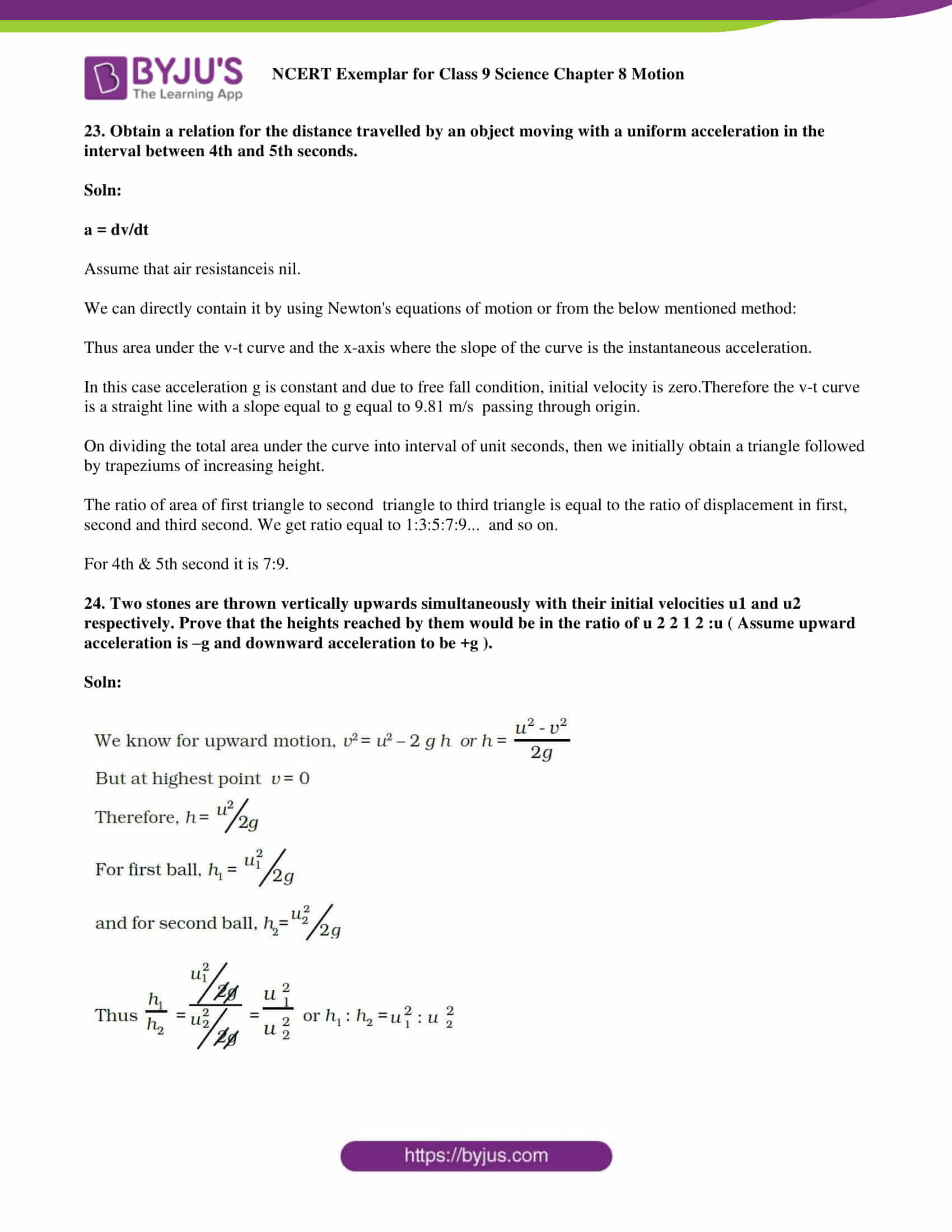### Multiple Choice Questions

1. A particle is moving in a circular path of radius r. The displacement after half a circle would be:

(a) Zero

(b) π r

(c) 2 r

(d) 2π r

Soln:

Explanation:

After half revolution

Distance travelled = X circumference= πr

Path length

Displacement = Final position- Initial Position

It comes out to be the diameter of the circle = 2R.

2. A body is thrown vertically upward with velocity u, the greatest height h to which it will rise is,

(a) u/g

(b) u2/2g

(c) u2/g

(d) u/2g

Soln:

Explanation:

V2= u2+2 as

here v = 0

a = -g

s = H

0 = u² -2gH

H = u²/2g

3. The numerical ratio of displacement to distance for a moving object is

(a) always less than 1

(b) always equal to 1

(c) always more than 1

(d) equal or less than 1

Soln:

Answer is (d) equal or less than 1

Explanation:

The shortest distance between the initial and the endpoint is called displacement. Distance is the total path length.

Displacement is vector and it may be positive or negative whereas Distance is scalar and it can never be negative.

The distance can be equal or greater than displacement which means the ratio of displacement to distance is always equal to or less than 1.

4. If the displacement of an object is proportional to square of time, then the object moves with

(a) uniform velocity

(b) uniform acceleration

(c) increasing acceleration

(d) decreasing acceleration

Soln:

Explanation:

Velocity is measured in distance /second and acceleration is measured in Distance/ second2. Hence Uniform acceleration is the right answer.

5. From the given v – t graph (Fig. 8.1), it can be inferred that the object is

(a) in uniform motion

(b) at rest

(c) in non-uniform motion

(d) moving with uniform acceleration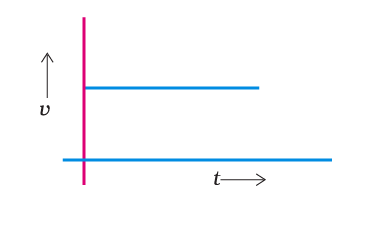Soln:

Answer is (a) in uniform motion

Explanation:

From the above-given graph, it is clear that velocity of the object remains constant throughout hence the object is in uniform motion.

6. Suppose a boy is enjoying a ride on a merry-go-round which is moving with a constant speed of 10 m/s. It implies that the boy is

(a) at rest

(b) moving with no acceleration

(c) in accelerated motion

(d) moving with uniform velocity

Soln:

Answer is (c) in accelerated motion

Explanation:

Boy is moving in a circular motion and circular motion is an accelerated motion hence C) is right answer.

7. Area under a v – t graph represents a physical quantity which has the unit

(a) m2

(b) m

(c) m3

(d) m s–1

Soln:

Explanation:

The area given in the graph represents Displacement and its unit is meter. Hence the answer is (b) m

8. Four cars A, B, C and D are moving on a levelled road. Their distance versus time graphs are shown in Fig. 8.2. Choose the correct statement

(a) Car A is faster than car D.

(b) Car B is the slowest.

(c) Car D is faster than car C.

(d) Car C is the slowest.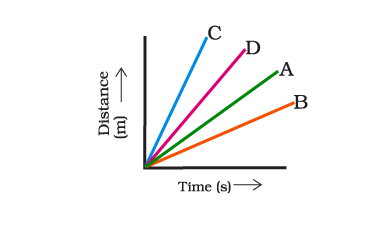Soln:

Answer is (b) Car B is the slowest.

Explanation:

Graph shows that Car B covers less distance in a given time than A, C and D cars hence it is the slowest.

9. Which of the following figures (Fig. 8.3) represents uniform motion of a moving object correctly?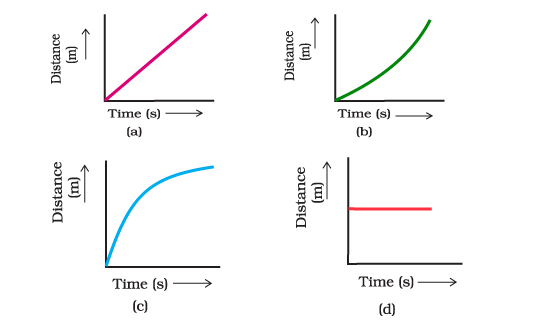Soln:

Explanation:

Distance in graph a) is uniformly increasing with time hence it represents uniform motion.

10. Slope of a velocity – time graph gives

(a) the distance

(b) the displacement

(c) the acceleration

(d) the speed

Soln:

11. In which of the following cases of motions, the distance moved and the magnitude of displacement are equal?

(a) If the car is moving on straight road

(b) If the car is moving in circular path

(c) The pendulum is moving to and fro

(d) The earth is revolving around the Sun

Soln:

Answer is (a) If the car is moving on a straight road

Explanation:

In other cases given here displace can be less than distance hence option (a) If the car is moving on a straight road is the right answer.

12. The displacement of a moving object in a given interval of time is zero. Would the distance travelled by the object also be zero? Justify your answer.

Soln:

Displacement zero does not mean zero distance. The distance can be zero when moving an object back to the place it started. Displacement is either equal or less than distance but the distance is always greater than one and it cannot be a negative value.

13. How will the equations of motion for an object moving with a uniform velocity change?

Soln:

If object is moving with a uniform velocity then v=µ and a=0. In this scenario equation for distance is given below.

S= ut and V2– µ2=0

14. A girl walks along a straight path to drop a letter in the letterbox and comes back to her initial position. Her displacement–time graph is shown in Fig.8.4. Plot a velocity-time graph for the same.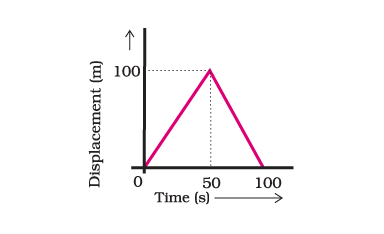Soln: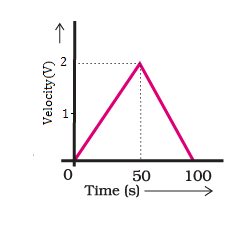15. A car starts from rest and moves along the x-axis with constant acceleration of 5 m/s2 for 8 seconds. If it then continues with constant velocity, what distance will the car cover in 12 seconds since it started from the rest?

Soln:

Car Starts from rest hence Initial velocity u=o acceleration a=5 m/s2 and time t=8s

v = u+at

v = 0+5×8

v = 40ms-1

From second equation

s = ut +

$$\begin{array}{l}\frac{1}{2}\end{array}$$
at2

s = 0x8 +

$$\begin{array}{l}\frac{1}{2}\end{array}$$
x5x(8)2

s =

$$\begin{array}{l}\frac{1}{2}\end{array}$$
x5x(8)2

s =

$$\begin{array}{l}\frac{1}{2}\end{array}$$
x5x64

s = 5×32=160 is the distance coveed in 8 seconds.

The total distance covered in 12 seconds, therefore, is 160+160=320m

16. A motorcyclist drives from A to B with a uniform speed of 30 km/h and returns back with a speed of 20 km h–1. Find its average speed.

Soln:

Let the distance from A to B is D kms.

Distance for the entire journey is 2D kms.

Time taken to go from A to B is D/30 hr and that of B to A is D/20 hr. So, total time taken T is

T = (D/30) + (D/20). By solving, we will get,

T = D/12 hrs.

Average speed = Total distance/Total time.

Av.speed = 2D ÷ D/12

=> 2D x 12/D = 24 km/h.

Hence Average speed of the motorcycle is 24 km/h.

17. The velocity-time graph (Fig. 8.5) shows the motion of a cyclist. Find (i) its acceleration (ii) its velocity and (iii) the distance covered by the cyclist in 15 seconds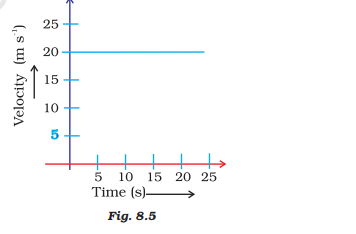Soln:

(i) As velocity is constant, acceleration is 0 m/s2

(ii) Here Velocity is constant hence v=20m/s

(iii) s = v x t

= 20 x 15

= 300 m

18. Draw a velocity versus time graph of a stone thrown vertically upwards and then coming downwards after attaining the maximum height.

Soln:

The velocity versus time graph of a stone thrown upwards vertically is as given below: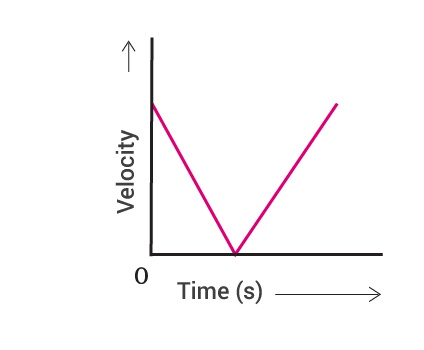19. An object is dropped from rest at a height of 150 m and simultaneously another object is dropped from rest at a height 100 m. What is the difference in their heights after 2 s if both the objects drop with same accelerations? How does the difference in heights vary with time?

Soln:

When two objects fall with the same acceleration simultaneously, after 2 seconds the difference in their heights will not change and it remains 50 m.Therefore the height of the first object after 2 seconds is 130 m.

In the same way, the height of the second object isTherefore, the height of the second object after 2 seconds is 80 m.

So, the difference is the same, i.e. 50 m.

This concludes that the difference in height of the two objects does not depend on time and will always be same.

20. An object starting from rest travels 20 m in first 2 s and 160 m in next 4 s. What will be the velocity after 7 s from the start.

Soln:

Here Object starts from rest hence initial velocity u=0 t =2s and s=20 m

According to Second equation of motion s= ut+at2

S = 0+

$$\begin{array}{l}\frac{1}{2}\end{array}$$
ax22

20 = 2+

$$\begin{array}{l}\frac{1}{2}\end{array}$$
ax22= 2a

= 20/2

a = 10m/s

According to first equation of motion velocity after 7 s from the start

V = u+at

V = 0+10×7

V = 70m/s

21. Using following data, draw time – displacement graph for a moving object:

 Time(s) 0 2 4 6 8 10 12 14 16 Displacement(m) 0 2 4 4 4 6 4 2 0

Use this graph to find average velocity for first 4 s, for next 4 s and for last 6 s.

Soln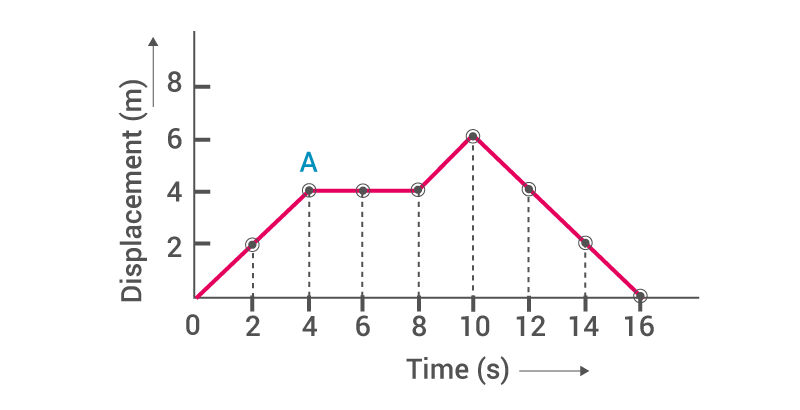Average velocity for the first 4s =

$$\begin{array}{l}\frac{change in displacement}{Total time taken}\end{array}$$
= (4-0)/(4-0)=4/4 = 1ms-1

Average velocity of next 4 s = V =

$$\begin{array}{l}\frac{4-4}{8-4}\end{array}$$
=0

Average velocity for last 6 s =

$$\begin{array}{l}\frac{(0-6)m}{(16-10)s}\end{array}$$
=
$$\begin{array}{l}\frac{-6}{6}\end{array}$$
= 1 ms-1

22. An electron moving with a velocity of 5 × 104 m/s enters into a uniform electric field and acquires a uniform acceleration of 104 ms-2 in the direction of its initial motion.

(i) Calculate the time in which the electron would acquire a velocity double of its initial velocity.

(ii) How much distance the electron would cover in this time?

Soln:

Given initial velocity, u = 5 × 104 m/s  and acceleration, a = 104ms-2

(i) final velocity = v = 2 u = 2 × 5 ×104 m/s =10 × 104 m/s

To find t,      use  v  =  at  or t = u – u / a = (5 × 104)/104

=5s

(ii)   Using s = ut +

$$\begin{array}{l}\frac{1}{2}\end{array}$$
at 2  = (5 ×104) × 5 +
$$\begin{array}{l}\frac{1}{2}\end{array}$$
(10 ) × (5) 2

= 25 ×104 + 25 /2 ×104

= 37.5×104 m

23. Obtain a relation for the distance travelled by an object moving with a uniform acceleration in the interval between 4th and 5th seconds.

Soln:

a = dv/dt

Assume that air resistance is nil.

We can directly contain it by using Newton’s equations of motion or from the below-mentioned method:

Thus area under the v-t curve and the x-axis where the slope of the curve is the instantaneous acceleration.

In this case acceleration, g is constant and due to free-fall condition, the initial velocity is zero. Therefore the v-t curve is a straight line with a slope equal to g equal to 9.81 m/s  passing through the origin.

On dividing the total area under the curve into the interval of unit seconds, then we initially obtain a triangle followed by trapeziums of increasing height.

The ratio of the area of the first triangle to second triangle to the third triangle is equal to the ratio of displacement in first, second and third second. We get ratio equal to 1:3:5:7:9…  and so on.

For 4th & 5th second it is 7:9.

24. Two stones are thrown vertically upwards simultaneously with their initial velocities u1 and u2 respectively. Prove that the heights reached by them would be in the ratio of u1² :u2²

( Assume upward acceleration is –g and downward acceleration to be +g ).

Soln: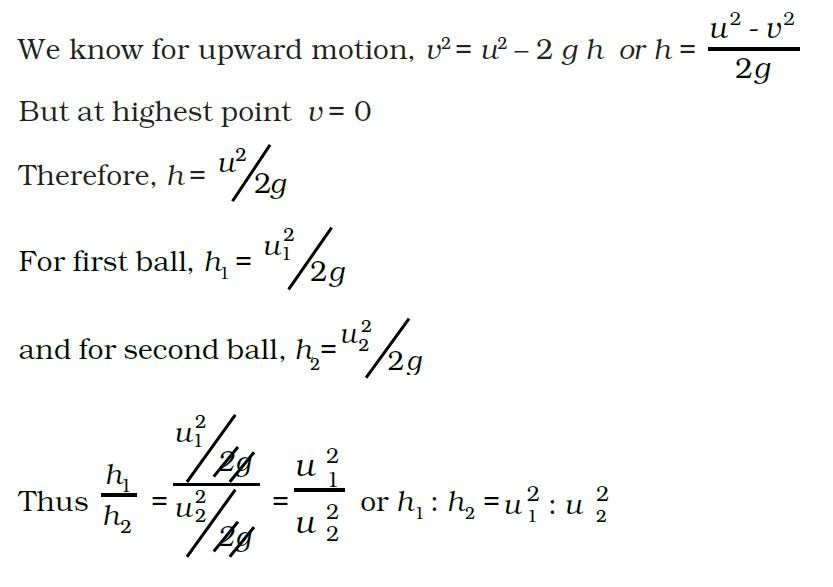### Important topics of NCERT Exemplar for Class 9 Science Chapter 8 Motion

• Introduction
• Speed
• Velocity
• Distance and displacement
• Uniform and non-uniform motion
• Equations of motion
• Uniform circular motion

We, at BYJU’S, provide NCERT Exemplar in a chapter-wise to help students in their studies. All the solutions in this study material are explained in a simple language and in an easy format for students to learn better and effectively. These NCERT Exemplars for Class 9 Science, Chapter 8 Motion provide complete information regarding the topic along with the definitions and examples. These NCERT Exemplars are available in PDF format and students can directly download these materials or can practise online by visiting our website at BYJU’S website or by downloading BYJU’S App. For CBSE study materials, notes, sample papers, videos and animation visit our website at BYJU’S or download the BYJU’S App.

## Frequently Asked Questions on NCERT Exemplar Solutions for Class 9 Science Chapter 8

### List out the topics discussed in the Chapter 8 of NCERT Solutions for Class 9 Science.

The topics discussed in the Chapter 8 of NCERT Solutions for Class 9 Science are –
1. Introduction
2. Speed
3. Velocity
4. Distance and displacement
5. Uniform and non-uniform motion
6. Equations of motion
7. Uniform circular motion.

### Explain the process of Uniform and non-uniform motion covered in Chapter 8 of NCERT Solutions for Class 9 Science.

Uniform motion is defined as the motion of an object in which the object travels in a straight line and its velocity remains constant along that line as it covers equal distances in equal intervals of time, irrespective of the duration of the time. Non uniform motion is defined as the motion of an object in which the object travels with varied speed and it does not cover the same distance in equal time intervals, irrespective of the time interval duration. Students who wish to understand the concepts covered in this chapter are advised to download the PDF of solutions from BYJU’S. The solutions are created by a set of highly experienced subject matter experts with the aim of helping students to score well in the board exams.

### Why is the NCERT Exemplar Solutions for Class 9 Science Chapter 8 important for the exams?

Class 9 is considered to be one of the crucial steps in students’ life. For this purpose, the faculty at BYJU’S have designed the chapter-wise solutions after conducting vast research on each concept. The solutions contain detailed and step wise explanations to help students score well in the board exams. PDF format of solutions is available on BYJU’S which can be downloaded and used by the students based on their requirements.